# opera package

#### 2016-08-17

The package opera provides several algorithm to perform robust online prediction of time series with the help of expert advice. In this vignette, we provide an example of how to use the package.

## Setting: when is the package opera useful?

Consider a sequence of real bounded observations $$y_1,\dots,y_n$$ to be predicted step by step. Suppose that you have at your disposal a finite set of methods $$k =1,\dots,K$$ (henceforth referred to as experts) that provide you before each time step $$t=1,\dots,n$$ predictions $$x_{k,t}$$ of the next observation $$y_t$$. You can form your prediction $$\hat y_t$$ using only knowledge of the past observations $$y_1,\dots,y_{t-1}$$ and past and current expert advice $$x_{k,1},\dots,x{k,t}$$ for $$k=1,\dots,K$$. The package opera implements several algorithms of the online learning literature that form predictions $$\hat y_t$$ by combining the expert advice according to there past performance. That is, $\hat y_t = \sum_{k=1}^K p_{k,t} x_{k,t} \,.$ These algorithms come with finite time worst-case guarantees. The monograph of Cesa-Bianchi and Lugisi (2006) gives a complete introduction to the setting of prediction of arbitrary sequences with the help of expert advice.

### What are the more important functions?

The package opera provides three important functions: mixture to build the algorithm object, predict to make a prediction by using the algorithm, and oracle to evaluate the performance of the experts and compare the performance of the combining algorithm.

## Example: predict the weekly electricity consumption.

Here, we provide a concrete example on how to use the package. To do so, we consider an electricity forecasting data set that includes weekly observations of the French electric load together with several covariates: the temperature, calendar information, and industrial production indexes. The data set is provided by the French National Institute of Statistics and Economic Studies (Insee).

### The data set

First, we load the data and we cut it into two subsets: a training set used to build the experts (base forecasting methods) and a testing set (here the last two years) used to evaluate the performance and to run the combining algorithms.

data(electric_load)
data_test <- electric_load[idx_data_test, ]  

The data is displayed in the following figures.

attach(electric_load)
plot(Load, type = "l", main = "The electric Load")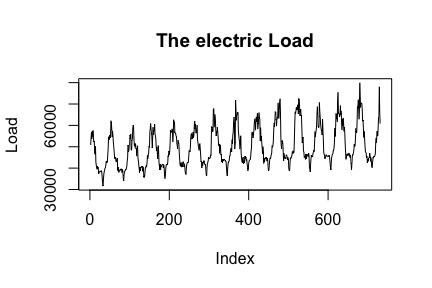plot(Temp, Load, pch = 16, cex = 0.5, main = "Temperature vs Load")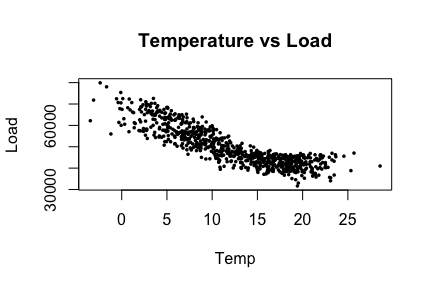plot(NumWeek, Load, pch = 16, cex = 0.5, main = "Annual seasonality")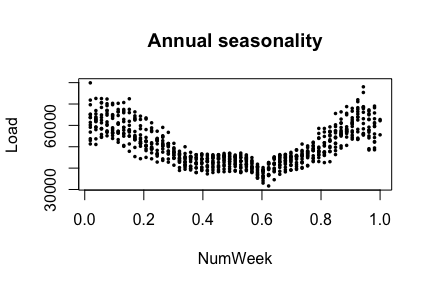### First: build the expert forecasts

Here, we build three base forecasting methods to be combined later.

• A generalized additive model using the mgcv package:
library(mgcv)
gam.fit <- gam(Load ~ s(IPI) + s(Temp) + s(Time, k=3) +
s(Load1) + as.factor(NumWeek), data = data_train)
gam.forecast <- predict(gam.fit, newdata = data_test)
• A medium term generalized additive model followed by an autoregressive short-term correction.
# medium term model
medium.fit <- gam(Load ~ s(Time,k=3) + s(NumWeek) + s(Temp) + s(IPI), data = data_train)
electric_load$Medium <- c(predict(medium.fit), predict(medium.fit, newdata = data_test)) electric_load$Residuals <- electric_load$Load - electric_load$Medium

# autoregressive correction
ar.forecast <- numeric(length(idx_data_test))
for (i in seq(idx_data_test)) {
ar.fit <- ar(electric_load$Residuals[1:(idx_data_test[i] - 1)]) ar.forecast[i] <- as.numeric(predict(ar.fit)$pred) + electric_load$Medium[idx_data_test[i]] } • A gradient boosting model using caret package library(caret) gbm.fit <- train(Load ~ IPI + IPI_CVS + Temp + Temp1 + Time + Load1 + NumWeek, data = data_train, method = "gbm") gbm.forecast <- predict(gbm.fit, newdata = data_test) Once the expert forecasts have been created (note that they can also be formed online), we build the matrix of expert and the time series to be predicted online Y <- data_test$Load
X <- cbind(gam.forecast, ar.forecast, gbm.forecast)
matplot(cbind(Y, X), type = "l", col = 1:6, ylab = "Weekly load", xlab = "Week", main = "Expert forecasts and observations")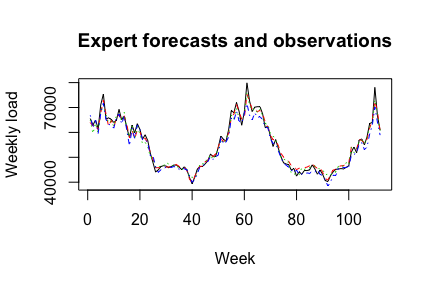### How good are the expert? Look at the oracles

To evaluate the performance of the experts and see if the aggregation rules may perform well, you can look at the oracles (rules that are used only for analysis and cannot be design online).

oracle.convex <- oracle(Y = Y, experts = X, loss.type = "square", model = "convex")
## Warning in bestConvex(Y, experts, awake = awake, loss.type = loss.type, :
## The best convex oracle is only approximated (using optim).
plot(oracle.convex)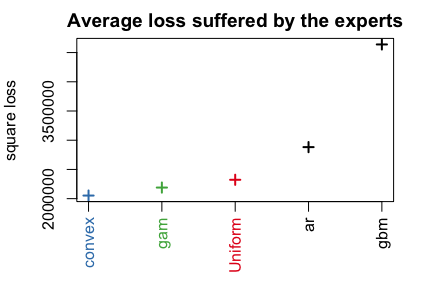print(oracle.convex)
## Call:
## oracle.default(Y = Y, experts = X, model = "convex", loss.type = "square")
##
## Coefficients:
##    gam    ar   gbm
##  0.715 0.153 0.131
##
##                       rmse   mape
## Best expert oracle:   1480 0.0202
## Uniform combination:  1520 0.0197
## Best convex oracle:   1430 0.0189

The parameter loss.type defines the evaluation criterion. It can be either the square loss, the percentage loss, the absolute loss, or the pinball loss to perform quantile regression.

The parameter model defines the oracle to be calculated. Here, we computed the best fixed convex combination of expert (i.e., with non-negative weights that sum to one).

### Aggregate the expert online using one of the possible aggregation procedures

The first step consists on initializing the algorithm by define the type of algorithm (Ridge regression, exponentially weighted average forecaster,$$\dots$$), the possible parameters, and the evaluation criterion. If no parameter is defined by the user, these will all be calibrated online by the algorithm. Bellow, we define the ML-Poly algorithm, evaluated by the square loss.

MLpol0 <- mixture(model = "MLpol", loss.type = "square")

Then, you can perform online predictions using the predict method. At each time, step the aggregation rule form a new prediction and update the procedure.

MLpol <- MLpol0
for (i in 1:length(Y)) {
MLpol <- predict(MLpol, newexperts = X[i, ], newY = Y[i])
}

The results can be displayed with method summary and plot.

summary(MLpol)
## Aggregation rule: MLpol
## Loss function:  square loss
## Coefficients:
##    gam    ar gbm
##  0.631 0.369   0
##
## Number of experts:  3
## Number of observations:  112
## Dimension of the data:  1
##
##         rmse   mape
## MLpol   1460 0.0189
## Uniform 1520 0.0197
plot(MLpol, pause = TRUE)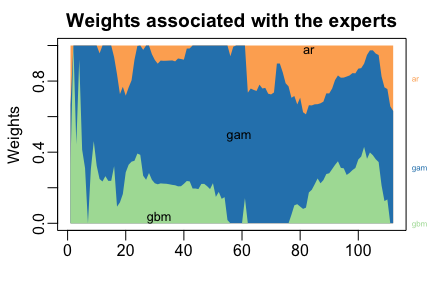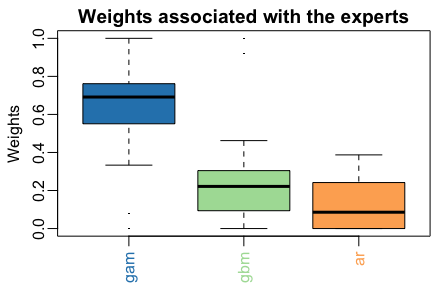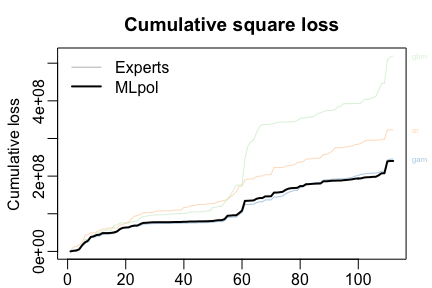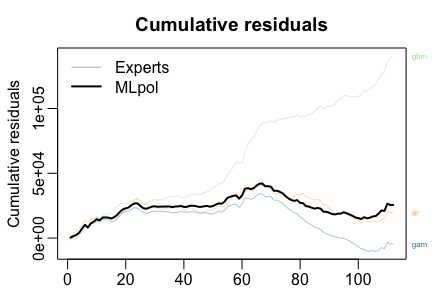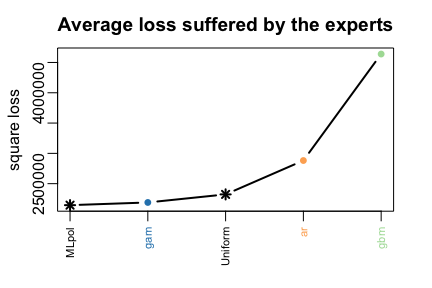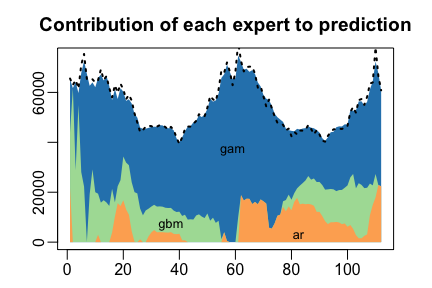The same results can be obtained more directly: * by giving the whole time series to predict specifying online = TRUE to perform online prediction.

MLpol <- predict(MLpol0, newexpert = X, newY = Y, online = TRUE)
• or directly to the function mixture, when building the aggregation rule
MLpol <- mixture(Y = Y, experts = X, model = "MLpol", loss.type = "square")